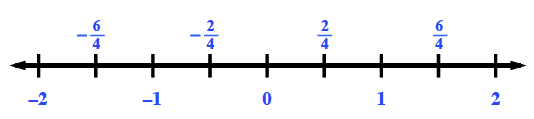### Home > CC1MN > Chapter 8 > Lesson 8.2.5 > Problem8-116

8-116.

Arrange the numbers below from least to greatest.

$\frac { 3 } { 5 }$, $\frac { 7 } { 3 }$$-\frac{3}{4}$, $1\frac { 2 } { 5 }$, $-\frac{1}{4}$

To begin, try determining which fractions or mixed numbers are less than or greater than $0$ and less than or greater than $1$.

It may also help to place the fractions in the general area they would fall on the number line below.
Can you place them in order from least to greatest now?The numbers from least to greatest are as follows:

$-\frac{3}{4}, -\frac{1}{4}, \frac{3}{5}, 1\frac{2}{5}, \frac{7}{3}$